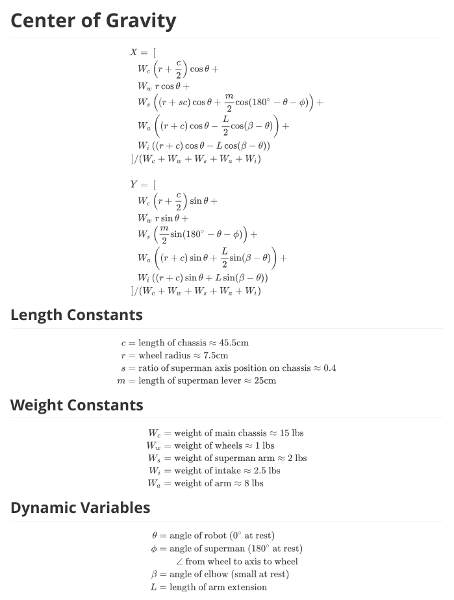# Center of Gravity calculations

Tags: Think and Control
Personhours: 2
Center of Gravity calculations By Arjun

### Task: Determine equations to find robot Center of GravityBecause our robot tends to tip over often, we decided to start working on a dynamic anti-tip algorithm. In order to do so, we needed to be able to find the center of gravity of the robot. We did this by modeling the robot as 5 separate components, finding the center of gravity of each, and then using that to find the overall center of gravity. This will allow us to better understand when our robot is tipping programmatically.

The five components we modeled the robot as are the main chassis, the arm, the intake, superman, and the wheels. We then assumed that each of these components had an even weight distribution, and found their individual centers of gravity. Finally, we took the weighted average of the individual centers of gravity in the ratio of the weights of each of the components.

By having equations to find the center of gravity of our robot, we can continuously find it programmatically. Because of this, we can take corrective action to prevent tipping earlier than we would be able to by just looking at the IMU angle of our robot.

### Next Steps

We now need to implement these equations in the code for our robot, so we can actually use them.

Date | March 30, 2019